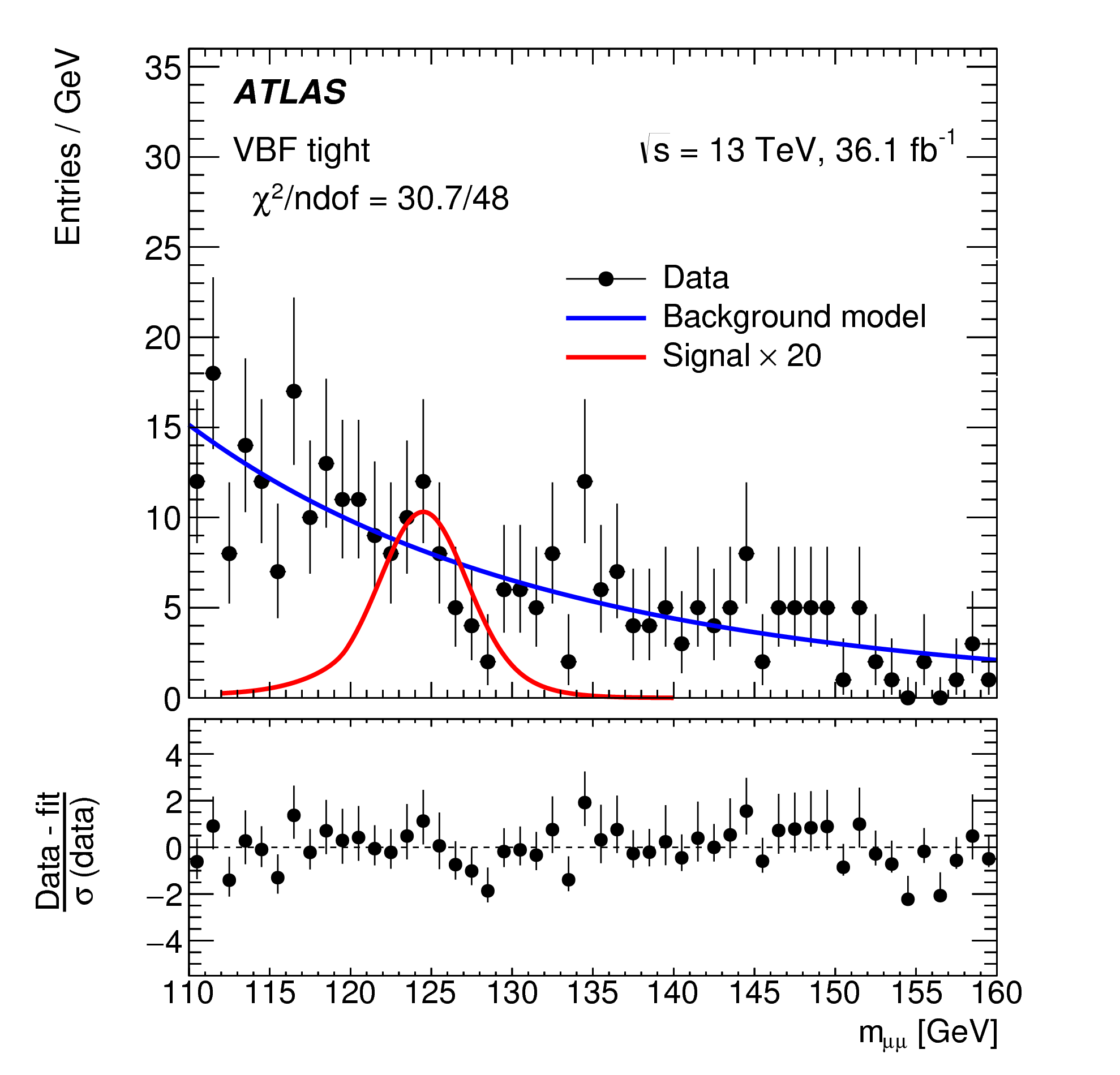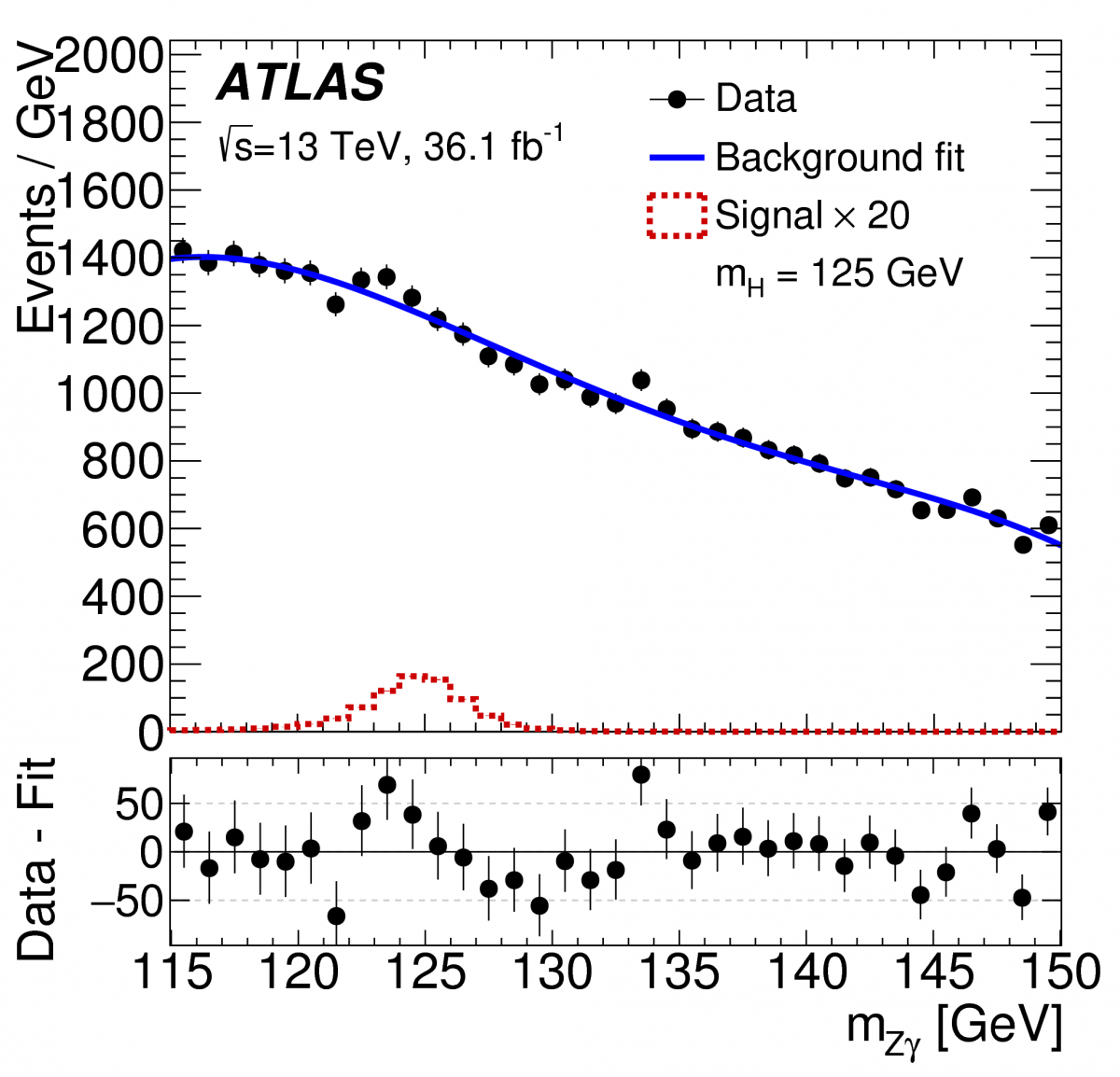Figure 1: The invariant mass of two muons from Higgs boson candidate decays, for the sub-category of events that has the best sensitivity to the Higgs signal. The red curve shows the prediction from the Standard Model Higgs boson with mass of 125 GeV multiplied by a factor of 20. The points with error bars are the data collected by ATLAS from the 2015 and 2016 dataset. The blue curve shows the result of a fit to the data using a background only model. The data are found to be fully consistent with the background model. (Image: ATLAS Collaboration/CERN)

Since discovering a Higgs boson in 2012, the ATLAS and CMS collaborations have been trying to understand whether this new particle is the Higgs boson predicted by the Standard Model, or a Higgs boson from a more exotic model containing new, as yet undiscovered, particles. The answer lies in the properties of the Higgs boson.

The Higgs boson mass has been measured to be 125.09 GeV with a precision of 0.2%. Using 2011 and 2012 data taken at 7 and 8 TeV proton–proton collision energy, respectively, the ATLAS experiment had studied some of the main processes through which the Higgs boson is produced, as well as its main decay channels (the Higgs boson is very short-lived and decays to known particles that are measured in the ATLAS detector). The results agree with Standard Model predictions, though within a fairly sizeable experimental uncertainty. These processes continue to be measured and improved with the 13 TeV Run 2 data, such as the recent first evidence for the decay of the Higgs boson to two bottom quarks.

To further our understanding of the properties of the Higgs boson, the ATLAS collaboration is also examining rare Higgs processes, including the Higgs boson decaying to two muons (H→μμ) and the Higgs boson decaying to a Z boson and a photon (H→Zγ). According to the Standard Model, only 0.022% and 0.15% of the Higgs bosons produced by ATLAS should decay to μμ or Zγ, respectively.

The ATLAS experiment has analysed 2015 and 2016 data taken at 13 TeV collision energy searching for these rare Higgs boson decays. In both decay channels, a potential signal would be visible as a narrow bump in the mass distribution of the decay particles over a smoothly falling background. Figure 1 shows this mass distribution observed in the μμ decay for the sub-category of events that has the best sensitivity to the Higgs signal. Figure 2 shows the mass distribution observed in the Zγ decay, with the Z reconstructed through its decay to a pair of electrons or muons. Neither show any hint of a Higgs boson signal yet.

### ATLAS has analysed 2015 and 2016 data taken at 13 TeV collision energy, searching for rare Higgs boson decays to two muons (H→μμ) and Higgs boson decays to a Z boson and a photon (H→Zγ).Figure 2: The invariant mass of the Z boson and photon from Higgs boson candidate decays. The red curve shows the prediction from the Standard Model Higgs boson with mass of 125 GeV multiplied by a factor of 20. The points with error bars are the data collected by ATLAS for the 2015 and 2016 dataset. The blue curve shows the result of a fit to the data using a background-only model. The data are found to be fully consistent with the background model. (Image: ATLAS Collaboration/CERN)

From these measured distributions we can determine how large a signal would have to be for us to see it clearly with the present dataset. We can then calculate the maximum amount of signal that can still hide in our data without us being able to clearly separate it from statistical fluctuations in the background. For the H→μμ decay, this is 2.8 times the expected amount of Standard Model Higgs bosons, when combined with measurements at 7 and 8 TeV. For the H→Zγ decay, it is 6.6 times the Standard Model prediction. With the present dataset, we would expect the limits to be 2.9 and 4.4 if the decays to two muons or to Zγ did not exist – thus the data are consistent with Standard Model predictions and limit exotic Higgs boson models that predict larger decay rates.

The fact that the Higgs boson decay to two muons has not been observed is an important result: it shows that the Higgs boson’s coupling to muons is much weaker than that to tau leptons. This is consistent with the Standard Model prediction that the Higgs boson couples to a particle with a strength proportional to the particle's mass and the tau lepton is 17 times heavier than the muon (see also ATLAS further verifies Standard Model coupling/mass relationship of Higgs boson).

So, while we do not yet have evidence of these rare decays, we are not far from Standard Model predictions. The much larger datasets expected over the coming years should allow the ATLAS experiment to discover these rare decays – if the Standard Model is correct! If the measured rates are found to be significantly different to predictions, then these rare channels will provide a glimpse into new physics processes beyond our present understanding.